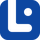# Articles related to "since"

### Subordinating Conjunctions

Subordinating conjunctions joins subordinate or dependent clauses to the main or independent clauses. To know all about these tricky grammatical words, click!

### Since

'Since' has different functions in the English language. In this lesson, we will discover more details about this word and learn when to use it.

### Since vs. Hence

'Since' and 'hence' talk about reasons and results. In this lesson, we will learn their similarities and differences.

### While vs. Since

While and since talk about time. That is why many learners confuse them. In this lesson, we will discover all about them.

### Since vs. After

'Since' and 'after' are used to express time. In this lesson, we will learn their similarities and differences.

### Compound-complex Sentences

A compound-complex sentence is comprised of at least two independent clauses and one or more dependent clauses. Let's get to know it in detail!

### Conjunctions of Cause and Effect

Conjunctions of cause and effect are used to express the reason or the result of an action. In this lesson, we will learn all about them.

### Conjunctions of Time

Conjunctions of time connect two clauses while stating the time. In this lesson, we will learn all about them.

### Because vs. Since

'Because' and 'since' express causes and effect but there is a distinction between them. In this lesson, we will learn their differences and similarities.

### For vs. Since

Both 'for' and 'since' are used to talk about how long an action continues. In this lesson, we will learn their uses and differences.

### For vs. Since

This time, we're gonna analyze two confusing grammatical prepositions. These two are widely used among native speakers.

### Once vs. Since

'Once' and 'since' are conjunctions but they are used in different contexts. In this lesson, we will learn their similarities and differences.

### Complex Sentences

A complex sentence is a sentence that contains an independent clause and one or more dependent clauses. In this lesson, we will learn all about this type!

### Since vs. Ever Since

'Since' and 'ever since' are used interchangeably when talking about a period of time. In this lesson, we will learn all about them.

### As vs. Since

'As' and 'since' are conjunctions of cause and effect. In this lesson, we will learn their similarities and differences.

### From vs. Since

'From' and 'since' are both used to talk about a specific time period. In this lesson, we will learn their uses and differences.

### Prepositions of Time

Prepositions allow us to talk about the relationship between two words in a sentence. Here, we will discuss the different prepositions of time in English.